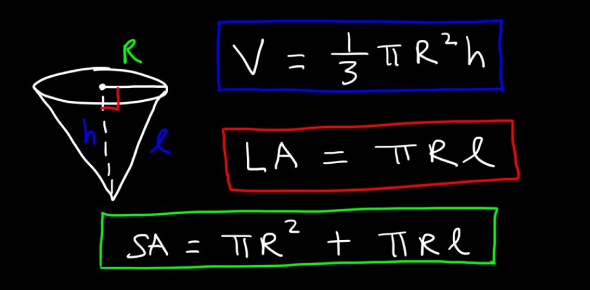# Volume And Surface Area Formulas

6 Questions | Attempts: 3964
ShareSettingsThis Quiz is about formulas of volume and surface area. Very easy quiz!

• 1.
What is the volume of a rectangular prism?
• A.

1/2 base x height

• B.

Length x base x height

• C.

Length x width

• 2.
What is the formula for the area of a trapazoid?
• A.

1/2 height ( b1+b2)

• B.

Base x height

• C.

Base x length

• 3.
What is the formula for the surface area of a rectangular prism?
• A.

3x lbh

• B.

Base x height

• C.

2lw +2wh + 2lh

• 4.
What is the volume for a triangular prism?
• A.

1/2 x base x height x length

• B.

1 x base x height

• C.

2 x base x length

• 5.
What is the formula for the surface area of cylinders?
• A.

A x b x c

• B.

2 x length x width

• C.

2π r h + 2π r²

• 6.
What is the formula for the volume of cylinders?
• A.

L x h

• B.

πr2h

• C.

B x h x l

## Related TopicsBack to top
×

Wait!
Here's an interesting quiz for you.